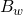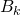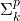Quantified Boolean Formulas

The next section in the appendix is the “Games and Puzzles” section.  But many of the reductions refer to this problem, so we’ll do this first.

The problem: Quantified Boolean Formulas (QBF).  This is problem LO11 in the appendix.

The description: Given a set U {u1..un} of variables, a formula F that consists of a quantifier Qi for each ui that is either ∀ or ∃, and a boolean expression E bound to the quantifiers, is F true?

Example: ∀ u1 ∀ u2 ∃ u3  (u1 ∨ u2 ∨ u3).  This is true because for each value of u1 and u2, we can find a value for u3 (namely “true”) that makes the expression true.

Reduction: G&J send you to the paper by Stockmeyer and Meyer that we did last week, and calls it a “generic transformation”, which means it builds a Turing Machine, similar to how Cook’s Theorem does.

But I think you can do a regular reduction from 3SAT: Given a SAT instance, we add a ∃ quantifier for each variable and then use the SAT formula as our E expression in this problem.  Then we’re just asking whether there exist values for each variable that make the formula true.

Difficulty: 3, assuming I’m not missing something.  I always worry when I find a “short cut” like this.

5 responses to “Quantified Boolean Formulas”

1.Gabriel Ferrer

Hi Sean,

I think your reduction is itself correct. That is, it is not difficult at all to show that QBF is NP-hard.

The more challenging issue is that QBF is not easily shown to be a member of NP. In fact, if I remember correctly QBF (also known as Q-SAT) is the foundational “hard” problem for PSPACE, and is actually PSPACE-Complete. So for it to be “merely” NP-Complete would require NP=PSPACE.

And that is what actually makes this interesting.

2.Sean T. McCulloch

That’s a good point- I should have mentioned the NP part. I wonder what the paper was actually trying to claim- I’ll have to check.

(And attempt to translate it from 1973 terminology into today’s)

3.Gabriel Ferrer

Also, it is no surprise that this kicks off the Games and Puzzles section. Lots of two-player game scenarios are PSPACE-Complete. Lots of those problems look something like this:

From the starting position, does there exist a move, such that for all opponent moves, does there exist a move, such that for all opponent moves…so that I am guaranteed to win?

This maps pretty nicely to the Quantified Boolean Formula concept. It’s just alternating there-exists and for-all quantifiers.

4.Sean T. McCulloch

Yeah, that’s basically what’ll be happening. Though some of them go through a lot of weird convolutions to set up the game in the way they want, so I guess it’s not trivial to set these things up.

For what it’s worth, Stockmeyer and Meyer’s claim is actually this:
Defineto be (I think) a QBF formula with w _sequences_ of variables, where we alternate between “There exists” and “For all” for each variable sequence. So w=1 is the Sat instance I talked about above.

They go on to state (but not really prove) thatis log-complete in. So basically it’s a way to get things all through the sigma hierarchy. Which is way more than I need here 🙂

5.Gabriel Ferrer

Right, that makes sense. Without universal (“for all”) quantifiers, the sequencing idea is not necessary.